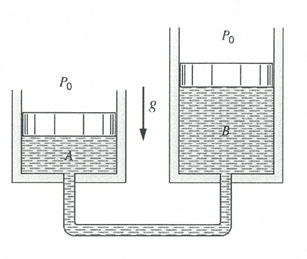## Piston Basics

Two cylinders containing a gas are connected as shown in the figure below. Piston A has a 5 cm diameter, while Piston B has a 3 cm diameter. The atmospheric pressure is 101 kPa.
1. If the mass of Piston A is 18 kg, what is the Piston B’s mass?
2. What is the pressure in the system?Hint
$$Pressure=\frac{Force}{Area}=\frac{mass\times acceleration}{area}$$$Hint 2 $$P_0+\frac{m_Ag}{A_A}=P_0+\frac{m_Bg}{A_B}$$$
where $$P_0$$ is the atmospheric pressure, $$m$$ is the mass, $$g$$ is the acceleration due to gravity, and $$A$$ is the area.
As a system, the pressures in Piston A and Piston B are equivalent. So,
$$P_A=P_B=\frac{Force}{Area}=\frac{mass\times acceleration}{area}$$$$$P_0+\frac{m_Ag}{A_A}=P_0+\frac{m_Bg}{A_B}$$$
where $$P_0$$ is the atmospheric pressure, $$m$$ is the mass, $$g$$ is acceleration due to gravity, and $$A$$ is the area. Solving for Piston B’s mass:
$$m_B=\frac{A_B}{A_A}m_A=\frac{3^2}{5^2}(18)=6.48\:kg$$$Solving for system pressure, $$P_A=P_B=P_0+\frac{m_Bg}{A_B}=101+\frac{(6.48)(9.81)(10)^{-3}}{\pi (0.03)^2/4}=190\:kPa$$$
1. 6.48 kg
2. 190 kPa